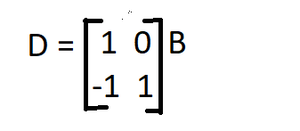# GATE | GATE CS 1996 | Question 58

Letbe two matrices such thatExpress the elements of D in terms of the elements of B.

Explanation:

As we know,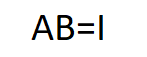we can clearly say,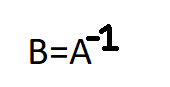Now,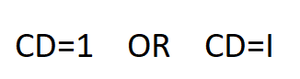write C in terms of A,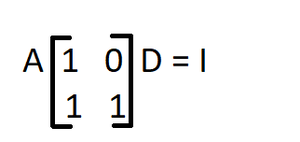Pre multiplying A-1 on both sides,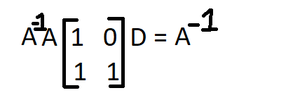As we know, A-1A = I,

Now, find inverse of the matrix which is multiplied with A then pre multiply the inverse of the matrix on both the sides,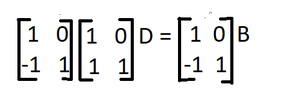As we know, multiplication of matrix and it’s inverse is identity matrix. So, D in terms of B becomes,